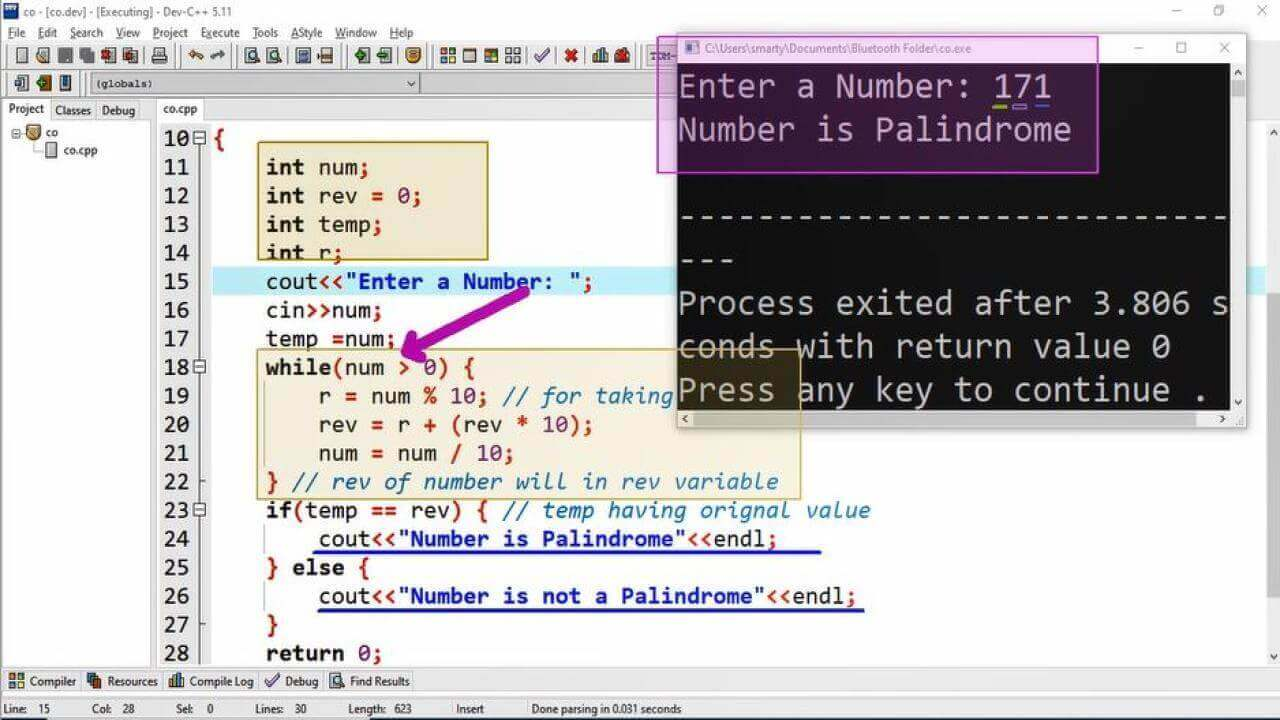# Program To Check Number Is Palindrome Or Not in CPP

## Source Code:

#include <iostream>
using namespace std;
/*
A number is palindrome if it's reverse is the number itself
1. Get reverse of a number
2. Compare reverse with orignal number
*/

int main(void)
{
int num;
int rev = 0;
int temp;
int r;
cout<<"Enter a Number: ";
cin>>num;
temp = num;
while(num > 0) {
r = num % 10; // for taking last digit of number
rev = r + (rev * 10);
num = num / 10;
} // rev of number will in rev variable
if(temp == rev) { // temp having orignal value
cout<<"Number is Palindrome"<<endl;
} else {
cout<<"Number is not a Palindrome"<<endl;
}
return 0;
}

## Output:## Working:

A number is called palindrome iff its reverse number is also its equivalent. In this C++ program we use while loop to get the reverse of given number. For this purpose we dclare three variable of int type. Here is the list of variable used in this program along with their use and data types.

 Sr. Variable Name Data Type Description 1 num int It is used to take input from the user. 2 rev int This variable will used to store the reverse of given number 3 temp int This temporary variable is used to store the input variable as its copy. 4 r int This variable is used in while loop for temporarily store remainder of number.
1. In this program we have use while loop that will repeat its statement until number is grater than or equal to zero. An the following steps are repeated.
2. Get remainder of number by 10
3. Calculate reverse using formula
4. Reduce the input number by 10

We use 10 to get remainder and to reduce number in while loop. Because the provide number belongs to Decimal number system. And the base/radix of decimal number system is 10. After the completion of while loop orignal number will be equal to 0 and reverse of input number will be in rev variable.

So finally we will compare the temp variable (as it contain value of input variable) with rev variable. If both are equal then we will print a message that given "Number is Palindrome" otherwise display a message "Number is not a Palindrome"